# DAX:SUMMARIZECOLUMNS 进阶

### IGNORE

SUMMARIZECOLUMNS函数本身会过滤所有度量值均为空的行，而IGNORE函数则可以令其忽略度量值的空值。如下：

``````SUMMARIZECOL =
SUMMARIZECOLUMNS (
'DimProductCategory'[ProductCategoryName],
'DimDate'[FiscalMonth],
"SALES", SUM ( 'FactSales'[SalesQuantity] )
)
``````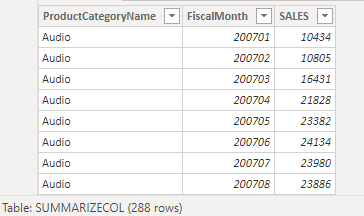``````SUMMARIZECOL_IGNORE =
SUMMARIZECOLUMNS (
'DimProductCategory'[ProductCategoryName],
'DimDate'[FiscalMonth],
"SALES", IGNORE(SUM ( 'FactSales'[SalesQuantity] ))
)
``````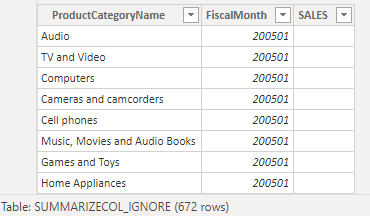### NONVISUAL

1. 该函数引用的必须是单个度量值
2. 度量值必须仅基于group-by列计算而得（本例中，即ProductCategoryName或FiscalMonth）

``````SUMMARIZECOL_NONVISUAL =
SUMMARIZECOLUMNS(
'DimProduct'[ProductName],
'DimProductCategory'[ProductCategoryName],
NONVISUAL(FIRSTNONBLANK('DimProductCategory'[ProductCategoryName],0)),
"SALES",SUM('FactSales'[SalesQuantity])
)
``````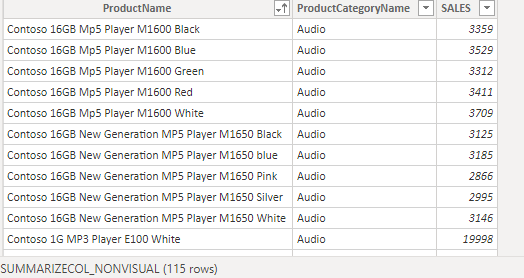``````SUMMARIZECOL_NONVISUAL =
SUMMARIZECOLUMNS(
'DimProduct'[ProductName],
'DimProductCategory'[ProductCategoryName],NONVISUAL(TREATAS({"TV and Video"},
'DimProductCategory'[ProductCategoryName])),
"SALES",SUM('FactSales'[SalesQuantity])
)
``````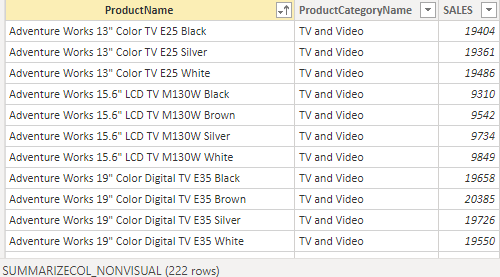``````ROLLUPADDISSUBTOTAL (
[<grandtotalFilter>],
<groupBy_columnName>,
<name>
[, [<groupLevelFilter>]
[, <groupBy_columnName>, <name> [, [<groupLevelFilter>] [, … ] ] ] ]
)
``````

• grandtotalFilter 为全局筛选，即决定哪些数据，参与小计的计算。
• groupLevelFilter 为组内筛选，即在每个小组内，对数据进行过滤，并返回过滤后的数据集以及其小计值。

``````SUMMARIZECOL_ROLLUP =
SUMMARIZECOLUMNS (
'DimProduct'[ProductName],
FILTER ( 'FactSales', 'FactSales'[ProductKey] > 100 ),
'DimDate'[Datekey],
"FILTER_DATE",
FILTER ( 'FactSales', 'FactSales'[DateKey] < DATE ( 2010, 1, 1 )
)
),
"SALES", SUM ( 'FactSales'[SalesQuantity] )
)
``````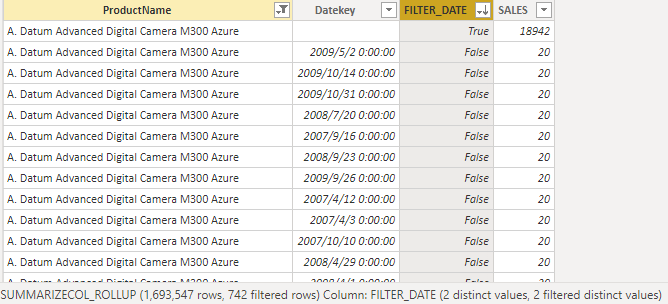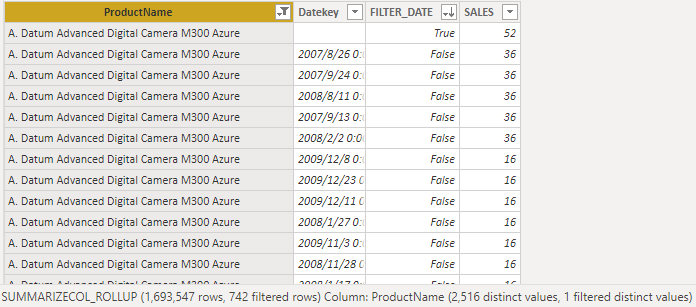### ROLLUPGROUP

``````SUMMARIZECOL_ROLLUP_WRONG =
SUMMARIZECOLUMNS (
'DimDate'[Datekey],'DimProduct'[ProductName] ,
FILTER ( 'FactSales', 'FactSales'[ProductKey] > 100 ),
'DimProduct'[ProductKey],
"IsProdTotal"
),
"SALES", SUM ( 'FactSales'[SalesQuantity] )
)
``````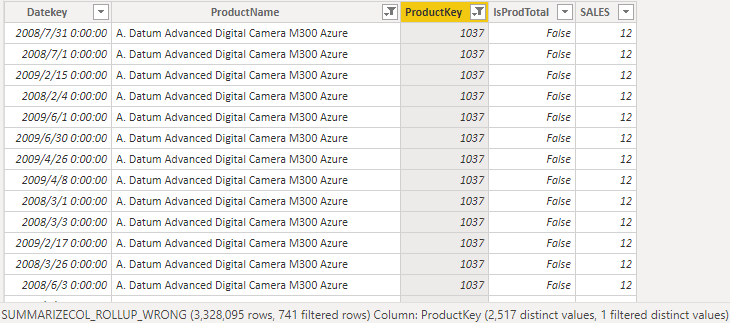ROLLUPGROUP就可用于解决此类问题。公式如下：

``````SUMMARIZECOL_ROLLUPGROUP =
SUMMARIZECOLUMNS (
'DimDate'[Datekey],
FILTER ( 'FactSales', 'FactSales'[ProductKey] > 100 ),
ROLLUPGROUP ( 'DimProduct'[ProductKey], 'DimProduct'[ProductName] ),
"IsProdTotal"
),
"SALES", SUM ( 'FactSales'[SalesQuantity] )
)
``````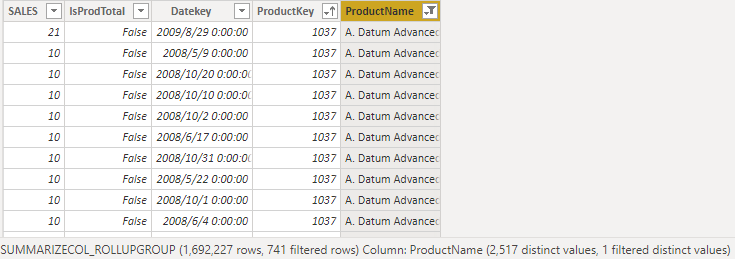### 总结